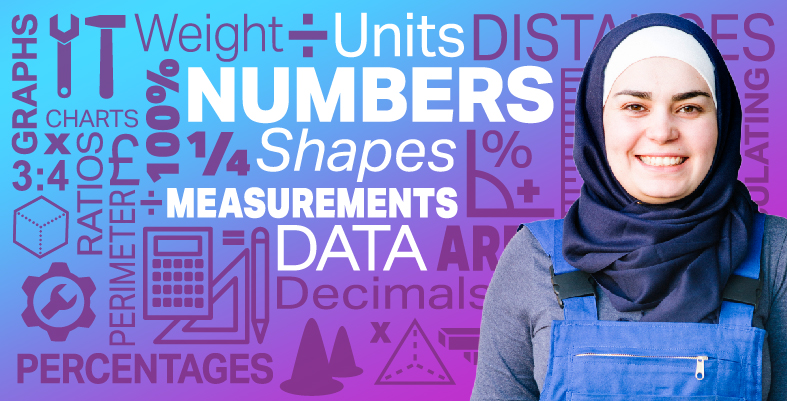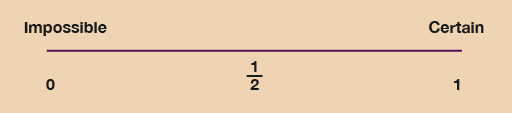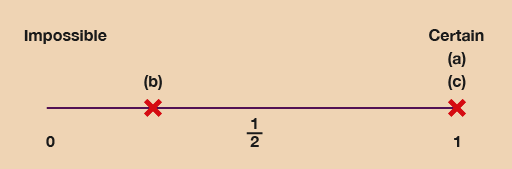Everyday maths for Construction and Engineering 1

Start this free course now. Just create an account and sign in. Enrol and complete the course for a free statement of participation or digital badge if available.

Free course

# 9.1 Probability scales

In real life, things usually fall somewhere in between the two extremes of ‘will never happen’ and ‘will definitely happen’.

We can use a probability scale to measure how likely events are to occur:Figure 13 A probability scale
• The probability of an impossible event (‘will never happen’) is 0.
• The probability of a certain event (‘will definitely happen’) is 1.
• All other events come between 0 and 1.
• Events with an even chance have a probability of .

Now try the following activity, where you’ll need a ruler and a pencil. Remember to check your answers once you have completed the questions.

## Activity 15: Looking at probability

Use a ruler to draw your own probability scale. Mark on it 0, 1/2 and 1. Label 0 as ‘impossible’ and 1 as ‘certain’.

Then mark these statements on the probability scale with crosses and label them with their question letter:

• a.The probability that the sun will rise tomorrow.
• b.The probability that you will compete in a Formula 1 car race next year.
• c.The probability of dying one day.Figure 14 A probability scale (answer)

Of course, if you are a racing car driver or plan to be one, your location for (b) might be closer to 1!

## Summary

In this section you have:

• learned about the possibility of different events happening
• shown that some events are more likely to occur than others.
FSM_SSC_1